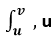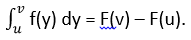Goseeko blog# What are the definite and indefinite integrals? Explained with examples

In calculus, the definite and indefinite integrals are the types of integration that we use to integrate the functions. The function can be exponential, power, logarithmic, constant, polynomial, or linear. We use these types of integral to determine the area under the curve.

The limits of calculus are also used to define the integral. In this post, we will learn the definition and formulas of the definite and indefinite integrals along with a lot of examples.

## What are definite and indefinite integrals?

The definite integral is a type of integral in which the upper and the lower limits are applied to integrate the functions. In this type of integral, the interval of initial and final terms of a graph are used. The interval (u, v) is also known as the boundaries of the function.

The equation used to define the definite integral is given below.

·       Inand v are the lower and upper boundaries respectively and it is known as the integral notation of definite integral.

·       f(y) is the integrand function.

·       dy is the variable of integral.

·       F(b) – F(a) is the fundamental rule of calculus to apply the boundaries.

·       M is the numerical value of the definite integral.

On the other hand, the indefinite integral is the other type of integral used to find the volume and area under the graph. In this type of integral, the upper and the lower limits are not applied. The simple integral notation is used in the indefinite integral.

ʃ f(y) dy = F(y) + C

·        ʃ it is the integral notation.

·       f(y) is the integrand function.

·       dy is the variable of integration.

·       F(y) is the result of the function after integrating the function.

·       C is the integral constant.

These types of integral are frequently used to find the area, volume, and central points.

### Rules of integration

Below are some well-known rules of integral.

## How to calculate the definite and indefinite integral?

By using the rules of integration, the problems of the definite and indefinite integral can be solved easily. Below are some examples of these types of integral.

Example 1: For the definite integral

Integrate 5x3 + 12sin(x) – 5x2y5 + 11x2 + 5 with respect to x have boundary values [1, 4].

Solution

Step 1: Use the integral notation of the definite integral and write the given function along with the variable of integration.

Step 2: Now use the sum rule and the difference rule of integral and apply the definite integral notation separately to each term.

Step 3: Use the constant and constant function rule of integral on the above expression.

Step 4: Now integrate the above expression by using the power and trigonometric rules of integral.

Step 5: Use the fundamental theorem of calculusTo avoid such a large number of steps to get the result, you can simply use an integral calculator. Follow the below step to integrate the function with the steps.

Step 1: Select the type of integral i.e., definite or indefinite.

Step 2: Input the integrand.

Step 3: choose the integrating variable.

Step 4: Enter the upper and lower limit in case of the definite integral.

Step 5: Press the calculate button to get the result.Step 6: The solution with steps will show below the calculate button.

Example 2: For indefinite integral

Integrate x5 – sin(x) – 5y5 + 13x3 + 5x with respect to x.

Solution

Step 1: Use the integral notation of the indefinite integral and write the given function along with the variable of integration.

ʃ (x5 – sin(x) – 5y5 + 13x3 + 5x) dx

Step 2: Now use the sum rule and the difference rule of integral and apply the indefinite integral notation separately to each term.

ʃ (x5 – sin(x) – 5y5 + 13x3 + 5x) dx = ʃ (x5) dx – ʃ sin(x) dx – ʃ 5y5 dx + ʃ 13x3 dx – ʃ 5x dx

Step 3: Use the constant and constant function rule of integral on the above expression.

ʃ (x5 – sin(x) – 5y5 + 13x3 + 5x) dx = ʃ (x5) dx – ʃ sin(x) dx – 5y5 ʃ dx + 13ʃ x3 dx – 5ʃ x dx

Step 4: Now integrate the above expression by using the power and trigonometric rules of integral.

ʃ (x5 – sin(x) – 5y5 + 13x3 + 5x) dx = x5+1 / 5 + 1 – (-cos(x)) – 5y5 (x) + 13 (x3+1 / 3 + 1) – 5 (x1+1 / 1 + 1) + C

ʃ (x5 – sin(x) – 5y5 + 13x3 + 5x) dx = x6 / 6 – (-cos(x)) – 5y5 (x) + 13 (x4 / 4) – 5 (x2 / 2) + C

ʃ (x5 – sin(x) – 5y5 + 13x3 + 5x) dx = x6/6 + cos(x) – 5xy5 + 13x4/4 – 5x2/2 + C

## Summary

Now you are witnessed that the types of the integral can be solved easily without any difficulty. In this post, we’ve learned about the basics of the types of integrals along with examples and also learned how to solve definite and indefinite integrals using an antiderivative calculator. Now you can solve any problem either by definite integral or indefinite easily.